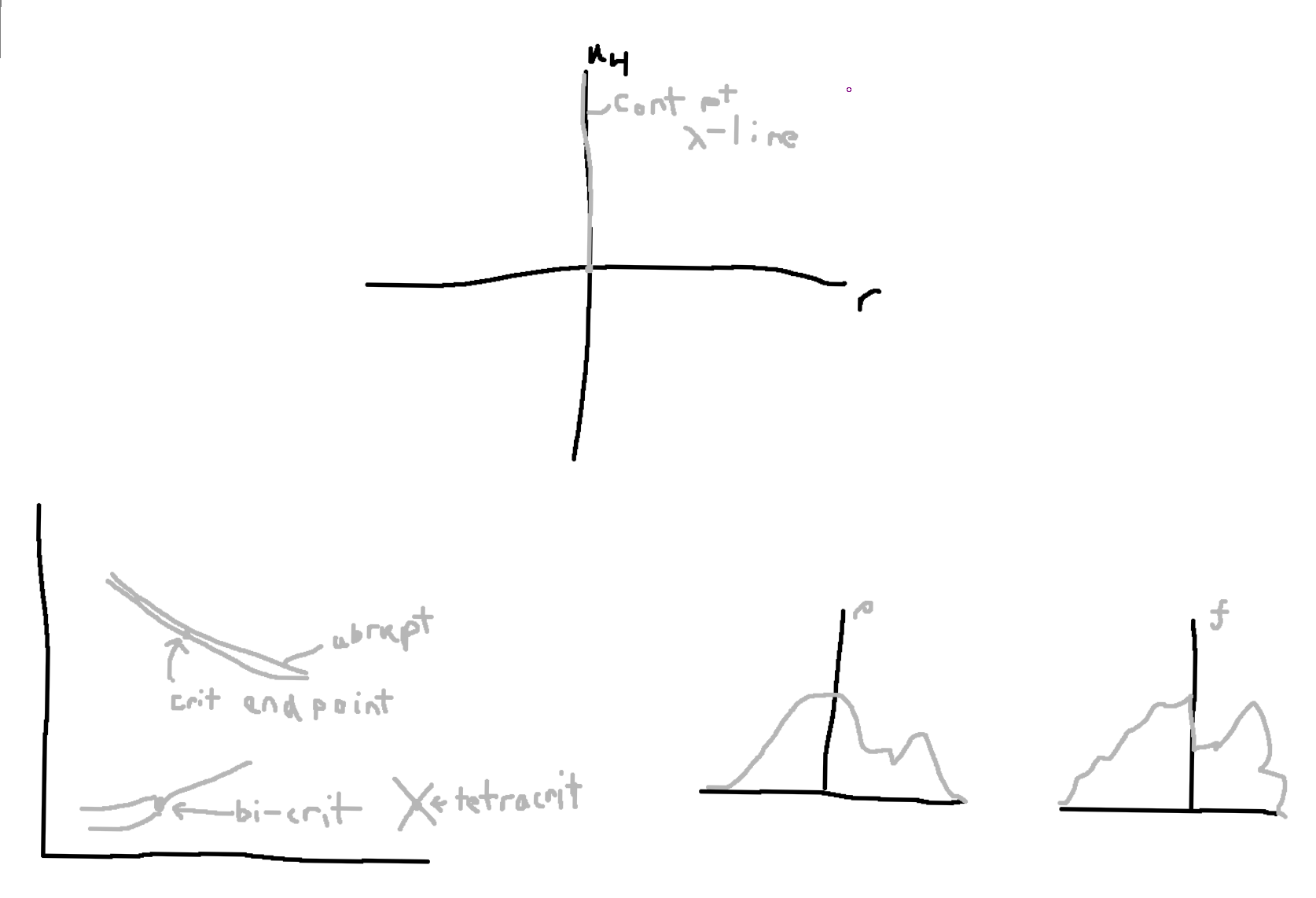# Correlations

$$C(\vec{r},\tau) = \langle \phi(\vec{x},t)\phi(\vec{x}+\vec{r},t+\tau)\langle$$.

Equal time correlation: $$C(\vec{r},\tau=0) = C(\vec{r}) = \langle\phi(\vec{x},t)\phi(\vec{x}+\vec{r},t)\rangle$$. Note this is averaged over both space and time, but there is no difference in time. $$\lim_{r\to\infty}C(\vec{r}) = C(\infty,0) = \langle \phi(\vec{x},t)\rangle\langle\phi(\vec{x}+\vec{r},t)\rangle$$ since they are independent and uncorrelated when far away. For our ising model, $$=m\cdot\ m = m^2$$.

Then, the connected correlation function, $$C^C(\vec{r},\tau) = \langle(\phi(\vec{x},t) - \langle\phi\rangle)(\phi(\vec{x}+\vec{r},t+\tau)-\langle\phi\rangle)\rangle$$, i.e. this only contains fully connected terms (c.f. Feynman diagram terms in the perturbation theory). In general, these can be measured. Example: X-ray scattering: $$I\propto |\rho_e(\vec{k})|^2$$ so

\begin{align} |\tilde{\rho}(\vec{k})|^2 &= \tilde{\rho}(\vec{k})^*\tilde{\rho}(\vec{k}) \nonumber \\ &= \int dx'\exp(i\vec{k}\cdot\vec{x}')\rho(\vec{x}')\int dx\exp(-i\vec{k}\cdot\vec{x})\rho(\vec{x}) \nonumber \\ &= \iint dx'dx\exp(i\vec{k}\cdot\vec{r})\rho(\vec{x}')\rho(\vec{x}) \nonumber \\ &= \int dr'\exp(i\vec{k}\cdot\vec{r})V\langle\rho(\vec{x}')\rho(\vec{x}'+ \vec{r})\rangle \nonumber \\ &= V\int dr'\exp(i\vec{k}\cdot\vec{r})C(\vec{r},0) \nonumber \\ &= V\tilde{C}(k). \end{align}

White noise implies uncorrelated sources.

$$C(r,\tau)$$ for an ideal gas. $$F^{id}(\rho(\vec{x}), T) = \rho kT \left(\ln(\rho\lambda^3)-1\right)$$.

So, for small fluctuations, $$F\approx F_0 + \mu_0(\rho-\rho_0) + \frac{1}{2}\alpha(\rho-\rho_0)^2$$ Then, integrating these we get $$\int Fdx \approx \frac{1}{2}\alpha(\rho-\rho_0) + \mathbb{C}$$. Thus, for small density fluctuations, $$F(\rho)\approx \frac{1}{2}\alpha(\rho-\rho_0)^2$$. Breaking up space to some boxes of $$\Delta V$$, $$P\sim \exp\left(-\frac{\frac{1}{2}\alpha(\rho-\rho_0)^2\Delta V}{kT}\right) = \exp\left(-\frac{(\rho-\rho_0)^2}{\frac{2}{\alpha\beta\Delta V}}\right)$$ Then, $$\sigma^{-2} = \alpha\beta\Delta V$$.

$$\langle(\rho-\rho_0)^2\rangle = \frac{(N-\langle N\rangle)^2}{(\Delta V)^2} = \frac{N}{(\Delta V)^2} = \frac{\rho_0}{\Delta V} = \frac{1}{\frac{\rho_0P_0}{\rho_0\rho_0P_0}\Delta V} = \frac{1}{\frac{P_0}{\rho_0P_0kT}\Delta V} = \frac{1}{\alpha\beta \Delta V}$$. $$C^{id}(\vec{r},0) = \begin{cases}0 & r>0\\ \frac{1}{\alpha\beta} & r=0 \\ \frac{\rho}{\Delta V} & \text{otherwise}\end{cases} = \frac{1}{\alpha\beta}\delta(\vec{r})$$. Hence, we get white noise, whose fourier transform is a flat distribution.

From last term, $$\sigma^2 \propto \sqrt{N}$$ and $$\frac{\sigma}{N}\sim \frac{1}{\sqrt{N}}$$. Then, $$p(m) \propto\exp\left(-\frac{m^2}{N}\right)$$.

Time evolution of densities $$\Rightarrow$$ diffusion equation. Time evolution of random density fluctuations$$(\rho_i)$$ $$\to \frac{\partial}{\partial t}\left(\rho(\vec{x},t)\right)_{\rho_i} = D\nabla^2\left(\rho(\vec{x},t)\right)_{\rho_i}$$ Onsager Regression Hypothesis.

Want: $$C^C(\vec{r},t) = \langle (\rho(\vec{x}+\vec{r},t+\tau) - \rho_0)(\rho(\vec{x},t) - \rho_0)\rangle_{ev}$$: averages $$\langle\cdot\rangle$$ ensemble average, no time dependence, $$\langle\cdot\rangle_{eq}$$ is equilibrium, $$\langle\cdot\rangle_{ev}$$ with time dependence, $$[\cdot]_i$$ noisy average over initial condition.

$$C^C(\vec{r},t) = \langle (\rho(\vec{x}+\vec{r},t+\tau) - \rho_0)(\rho(\vec{x},t) - \rho_0)\rangle_{ev}$$: Use translational invariance in space and time, thus we can set $$t$$ and $$\vec{x}$$ to zero. So, $$C^C(\vec{r},t) = \langle (\rho(\vec{r},\tau) - \rho_0)(\rho(0,0) - \rho_0)\rangle_{ev}$$

$$f[\rho(x)] = f_0 + \frac{\delta f}{\delta\rho}_{\rho_0} (\rho-\rho_0) + \frac{1}{2}\frac{\delta^2f}{\delta\rho^2}_{\rho_0}(\rho-\rho_0)^2$$.

$$\frac{\delta f}{\delta\rho} = \frac{\partial f}{\partial\rho} = \mu$$.

$$\frac{\delta^2 f}{\delta\rho^2} = \frac{\delta\mu}{\delta\rho} = \frac{\partial}{\partial\rho}(kT\ln\rho + 3kT\ln\lambda) = \frac{kT}{\rho_0} = \frac{P}{\rho_0^2}$$.

$$\int f[\rho]dx = V\mathcal{F}_0 + \mu \int(\rho-\rho_0)dx + \frac{1}{2}\int\alpha(\rho-\rho_0)^2dx \Rightarrow \mathcal{F}_{eff}[\rho] = \frac{1}{2}\alpha(\rho-\rho_0)^2$$.

So, $$p(\rho) \propto\exp(-\beta\int\frac{\alpha}{2}(\rho-\rho_0)^2dx)$$ Then for a some small volume, $$p_j(\rho)\propto \exp(-\beta\alpha(\rho-\rho_0)^2\Delta V/2)$$. From this, we wanted $$C(r,\tau) = \langle(\rho(x+r,t+\tau)-\rho_0)(\rho(x,t)-\rho_0)\rangle$$. Since we have a translationally invariant system, and we set time to zero, we get

\begin{align} C(r,0) &= \langle(\rho(r,\tau)-\rho_0)(\rho(0)-\rho_0)\rangle_{ev} \nonumber \\ &= \langle([\rho(r,\tau)]_i-\rho_0)(\rho_i(0)-\rho_0)\rangle_{eq}. \end{align}

Time evolving,

\begin{align} \frac{\partial C}{\partial t} &= \langle(\frac{\partial}{\partial t}[\rho]_i-\rho_0)(\rho_i(0)-\rho_0)\rangle \nonumber \\ &= \langle(D\nabla^2[\rho]_i-\rho_0)(\rho_i(0)-\rho_0)\rangle \nonumber \\ &= D\nabla^2\langle([\rho]_i-\rho_0)(\rho_i(0)-\rho_0)\rangle \nonumber \\ &= D\nabla^2C(\overline{r},t). \end{align}

$$C^{id}(r,\tau=0) = \frac{1}{\beta\alpha}\delta(\vec{r})$$. Then, $$\frac{1}{\beta\alpha}G(\vec{r},\tau) = \frac{1}{\beta\alpha}\left(\frac{1}{\sqrt{4\pi D\tau}}\right)^3\exp\left(-\frac{r^2}{4D\tau}\right)$$.Created: 2023-06-25 Sun 02:29

Validate